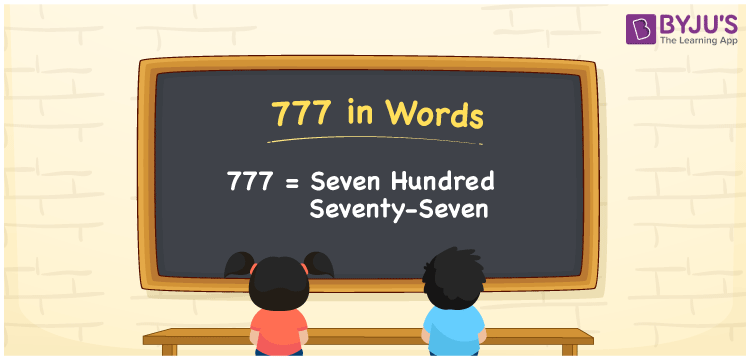# 777 in Words

777 in words is written as Seven hundred seventy-seven. In both the International System of Numerals and the Indian System of Numerals, 777 is written as Seven hundred seventy-seven. The number 777 is a Cardinal Number as it represents some quantity. For example, the “cost of the dress is 777 rupees”.

 777 in Words Seven hundred seventy-seven Seven hundred seventy-seven in Number 777

## 777 in English Words

We write 777 in English Words using the letters of the English alphabet. Therefore, we read 777 in English as “Seven hundred seventy-seven”.## How to Write 777 in Words?

To write 777 in words, we shall use the place value chart. In the place value chart, write 7 in the hundreds, tens, and ones, respectively. Now let us make a place value chart to write the number 777 in words.

 Hundreds Tens Ones 7 7 7

Thus, we can write the expanded form as

7 × Hundred + 7 × Ten + 7 × One

= 7 × 100 + 7 × 10 + 7 × 1

= 700 + 70 + 7

= 777

= Seven hundred seventy-seven.

777 is a natural number, the successor of 776 and the predecessor of 778.

777 in words – Seven hundred seventy-seven

• Is 777 an odd number? – Yes
• Is 777 an even number? – No
• Is 777 a perfect square number? – No
• Is 777 a perfect cube number? – No
• Is 777 a prime number? – No
• Is 777 a composite number? – Yes

## Frequently Asked Questions on 777 in Words

Q1

### How to write 777 in words?

777 in words is written as Seven hundred seventy-seven.
Q2

### How to write 777 in the International and Indian System of Numerals?

In both, the system of numerals, 777 in words, is written as Seven hundred seventy-seven.
Q3

### What is the successor of 777?

The successor of 777 is 778.